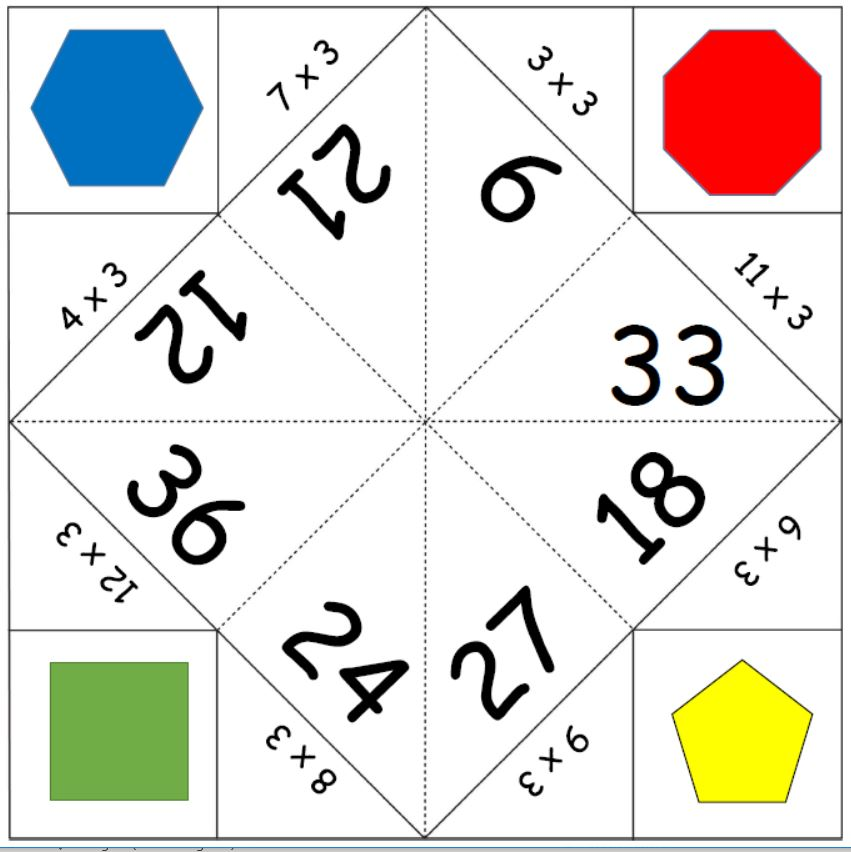# Easter themed maths word problems### Easter themed maths word problems

Free printable with maths words problems linked to Easter.  Includes multiplication,...

# Mr Men timetables word problems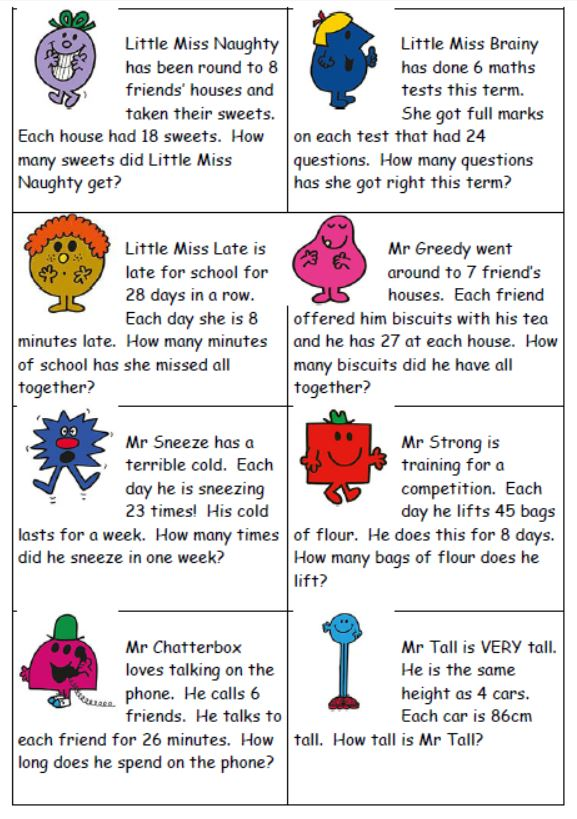### Mr Men timetables word problems

Free printable with 8 word problems for timestables in a Mr Man theme. ...

# Which strategy maths cards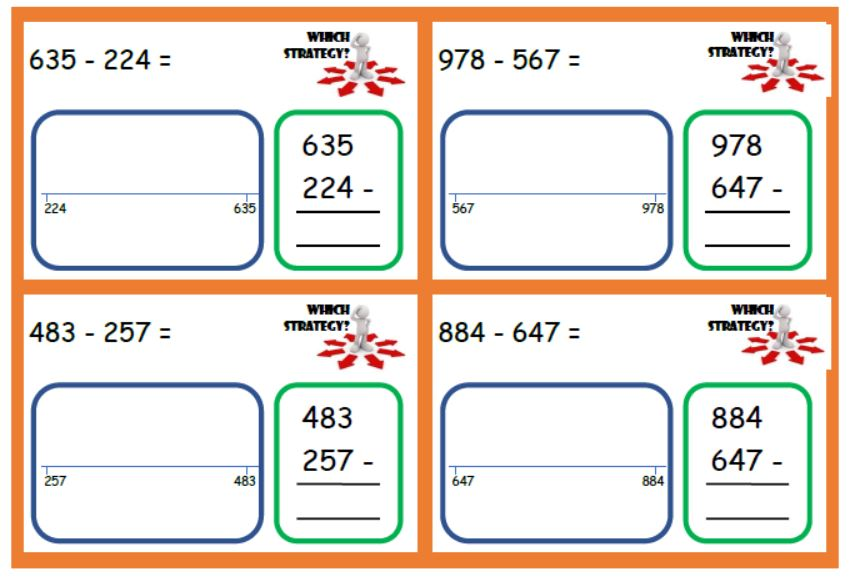### Which strategy maths cards

Free download to help children see that they can solve maths problems in a variety...

# Divide by 12 Fortune Teller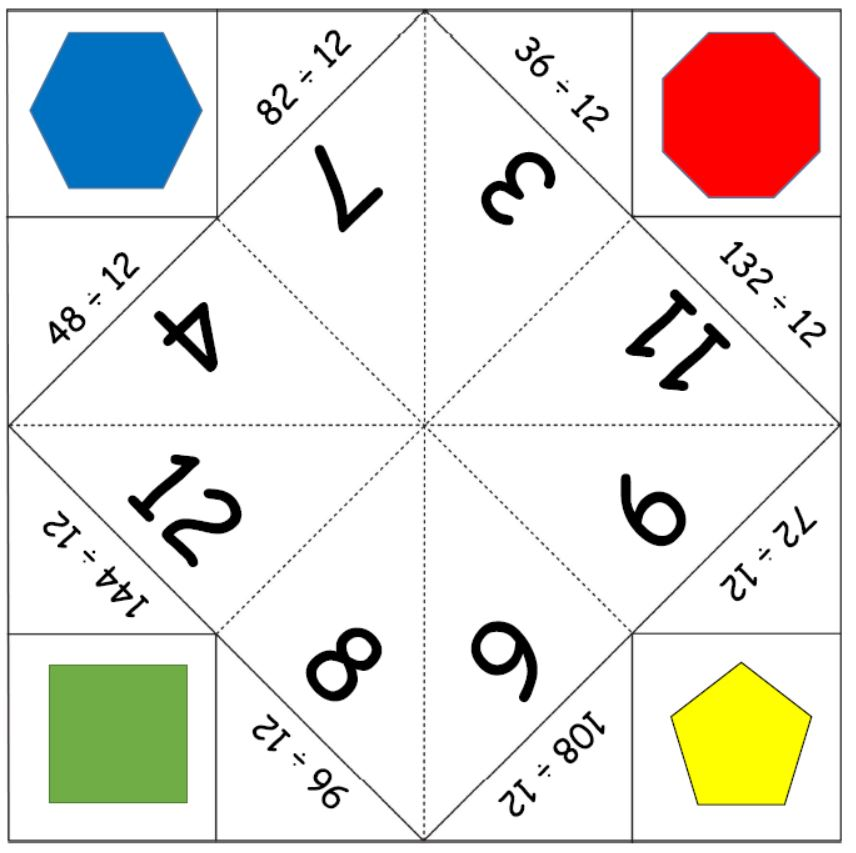### Divide by 12 Fortune Teller

A fun way to practise times tables- a free printable for the divide by 12 tables...

# Divide by 9 Fortune Teller### Divide by 9 Fortune Teller

A fun way to practise times tables- a free printable for the divide by 9 tables to...

# Divide by 8 Fortune Teller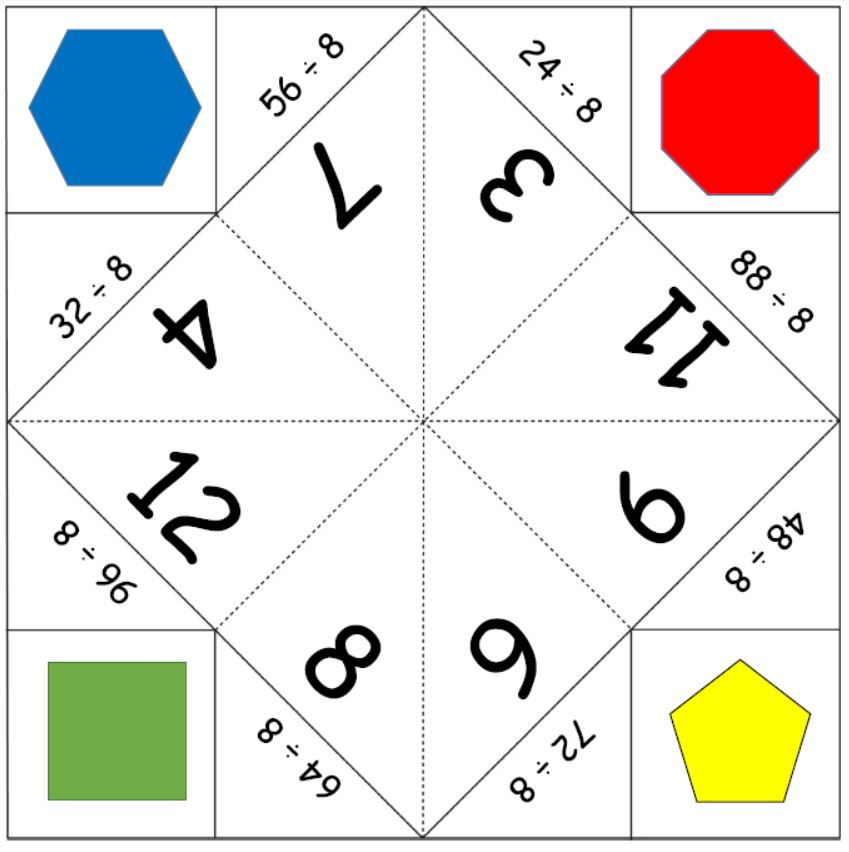### Divide by 8 Fortune Teller

A fun way to practise times tables- a free printable for the divide by 8 tables to...

# Divide by 7 Fortune Teller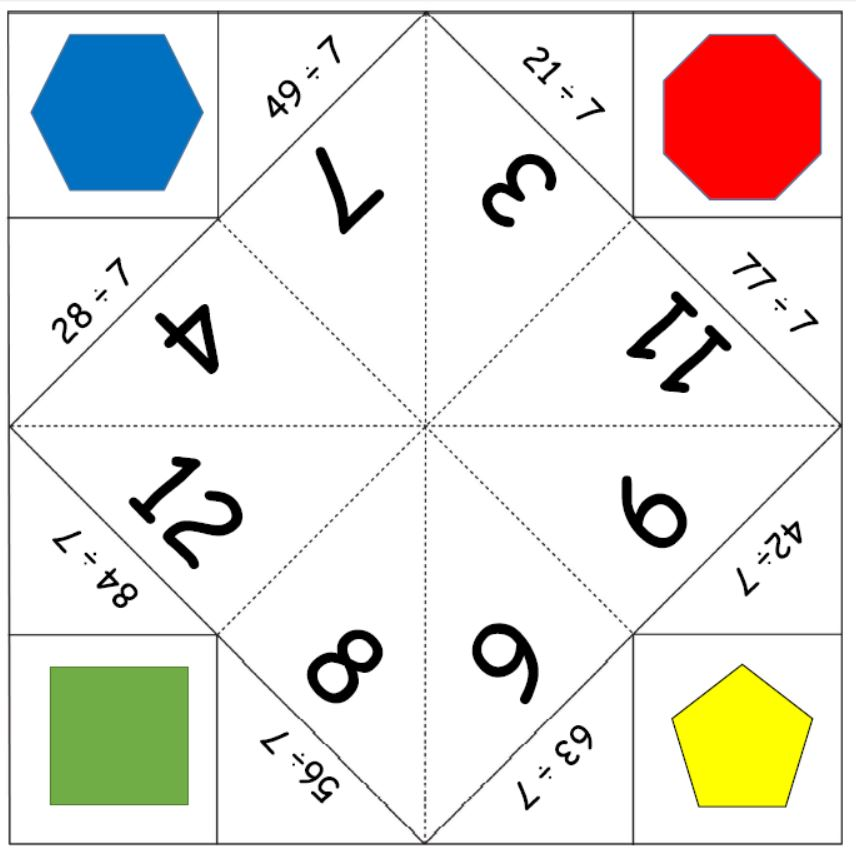### Divide by 7 Fortune Teller

A fun way to practise times tables- a free printable for the divide by 7 tables to...

# Divide by 6 Fortune Teller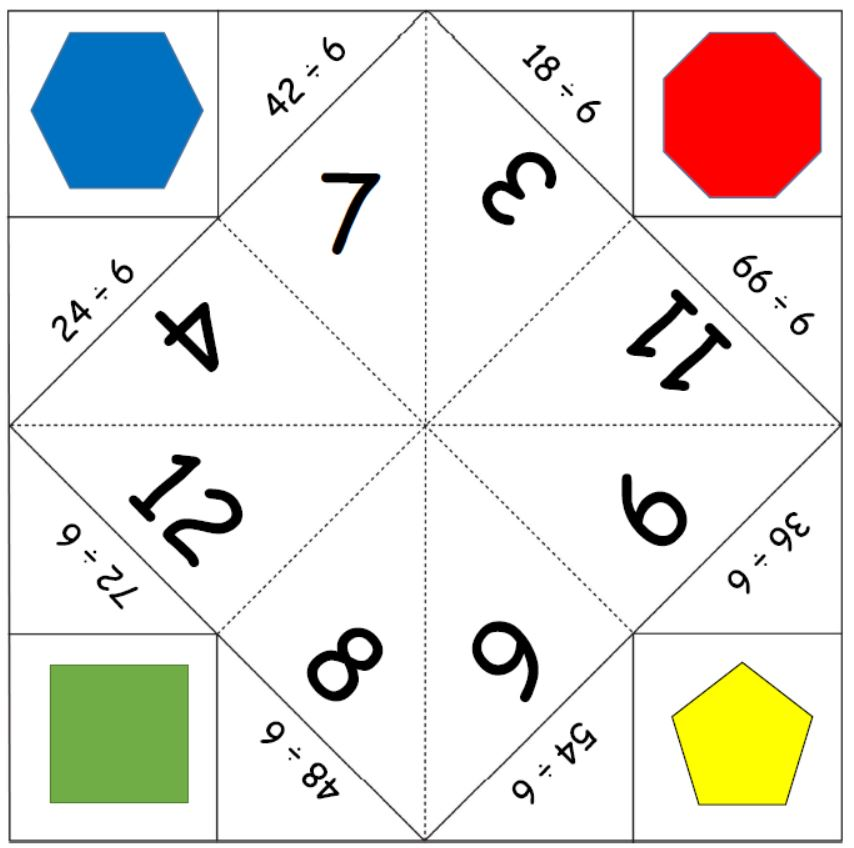### Divide by 6 Fortune Teller

A fun way to practise times tables- a free printable for the divide by 6 tables to...

# Divide by 5 Fortune Teller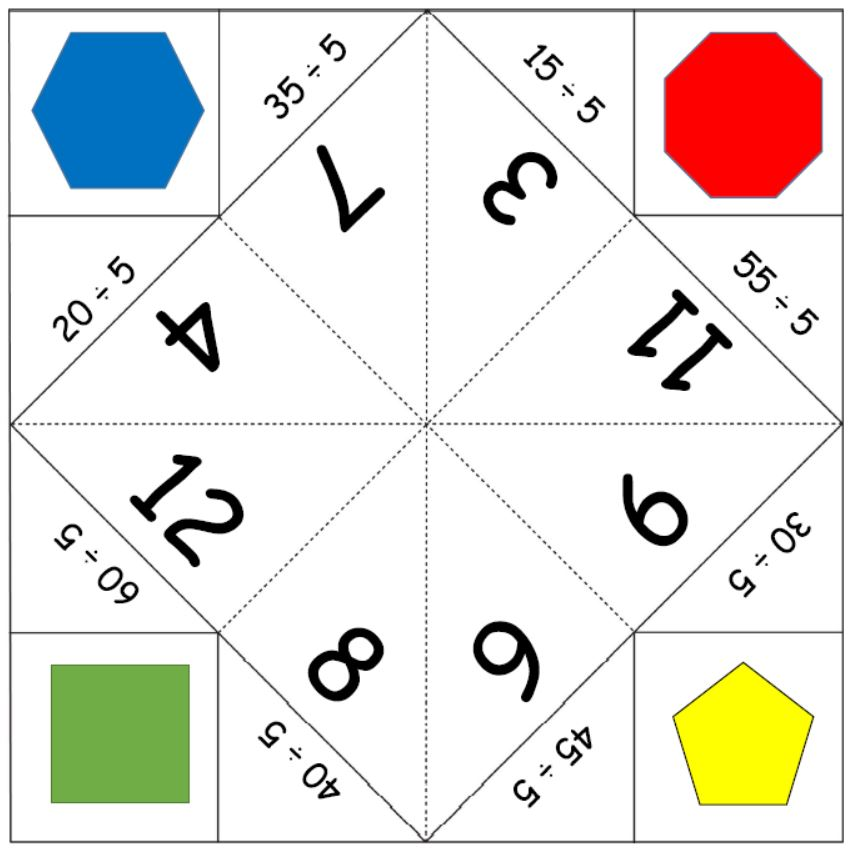### Divide by 5 Fortune Teller

A fun way to practise times tables- a free printable for the divide by 5 tables to...